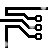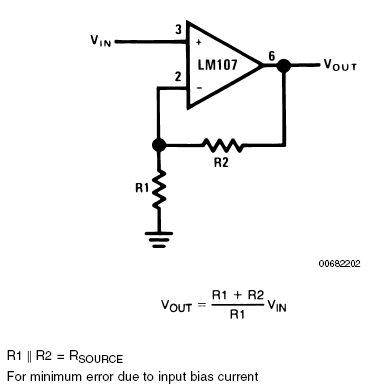## Non-Inverting Amplifier

### By internum

The Non-Inverting Amplifier, a high input impedance non-inverting circuit.

Figure 1 shows a high input impedance non-inverting circuit. This circuit gives a closed-loop gain equal to the ratio of the sum of R1 and R2 to R1 and a closed-loop 3 dB bandwidth equal to the amplifier unity-gain frequency divided by the closed-loop gain.Figure 1. Non-Inverting Amplifier

The primary differences between this connection and the inverting circuit are that the output is not inverted and that the input impedance is very high and is equal to the differential input impedance multiplied by loop gain. (Open loop gain/Closed loop gain.) In DC coupled applications, input impedance is not as important as input current and its voltage drop across the source resistance.

Applications cautions are the same for this amplifier as for the inverting amplifier with one exception. The amplifier output will go into saturation if the input is allowed to float. This may be important if the amplifier must be switched from source to source. The compensation trade off discussed for the inverting amplifier is also valid for this connection.1858509 December 2007